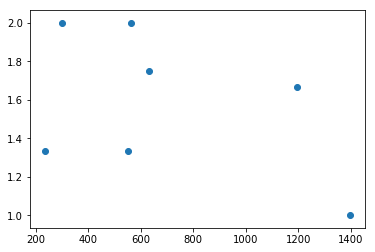## August 28, 2018

### Some observations of doing a bit of data analysis with DBSCAN and pandas in a Jupyter notebook

Sorting Classifications for making graphs-VolunteerClassifications
Here is a Jupyter notebook I was using today to parse the classifications from the Steelpan Vibrations project. I'm leaving some of the notes here as a reminder to myself for the future. (I learned how to put the Jupyter notebook into the blog from this page.)

I really want to share this because in all my reading on using DBSCAN to do cluster analysis, I had a hard time finding any page online that was describing how the coordinates of the points identified in a cluster could be paired with matched data from the larger (original) data set. When I found the solution (see link in the comments between cells below) it was really obvious, but it was painful not knowing even how to google for what I was looking for.

Function to do the cluster identification with DBSCAN:
In :
def dbscan(crds):
bad_xy = []  #might need to change this
X = np.array(crds)
db = DBSCAN(eps=18, min_samples=3).fit(X)
labels = db.labels_

n_clusters_ = len(set(labels)) - (1 if -1 in labels else 0)
unique_labels = set(labels)

colors = plt.cm.Spectral(np.linspace(0, 1, len(unique_labels)))

for k, col in zip(unique_labels, colors):
if k == -1:
# Black used for noise.
col = 'k'

# These are the definitely "good" xy values.
plt.plot(xy[:, 0], xy[:, 1], 'o', markerfacecolor=col,
markeredgecolor='k', markersize=14)
#print("\n Good? xy = ",xy)
#print("X = ",X)
# These are the "bad" xy values. Note that some maybe-bad and maybe-good are included here.
plt.plot(xy[:, 0], xy[:, 1], 'o', markerfacecolor=col,
markeredgecolor='k', markersize=6)

plt.title('Estimated number of clusters: %d' % n_clusters_)
plt.xlim(0, 512)
plt.ylim(0, 384)

clusters = [X[labels == i] for i in range(n_clusters_)]
#print(clusters)
#print(db.labels_)

return clusters, labels

Import the classifications into a pandas DataFrame. I'm using header=None because there were no headings in the csv file:
In :
import pandas as pd

This is the main part of the code that ends up calling the dbscan function at the end:
In :
from matplotlib.patches import Ellipse
import matplotlib.pyplot as plt
import matplotlib.cm as cm
import matplotlib.colors as col
cmap_1 = cm.ScalarMappable(col.Normalize(1, 11, cm.gist_rainbow))
import numpy as np
from sklearn.cluster import DBSCAN

x_val = []
y_val = []
frng = []
crds = []
ell = []

for centers in df.values:
x_val.append(centers)
y_val.append(centers)
frng.append(centers)
crds.append([centers, centers])
ell.append(Ellipse(xy=[centers, centers], width=centers, height=centers, angle=centers))
centers_raw = {'XVal': x_val,
'YVal': y_val,
'Fringe': frng}

centers_df = pd.DataFrame(centers_raw, columns=['XVal', 'YVal', 'Fringe'])
plt.figure(0)
plt.scatter(centers_df.XVal, centers_df.YVal, s=20, c=cmap_1.to_rgba(centers_df.Fringe), alpha=.6)
plt.xlim(0, 512)
plt.ylim(0, 384)
#plt.title('Subject id = %s'%(coords_x))
plt.show()
#print(crds)
plt.figure(1)
clusters, labels = dbscan(crds)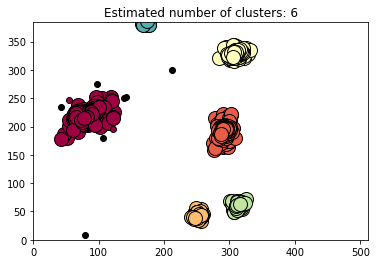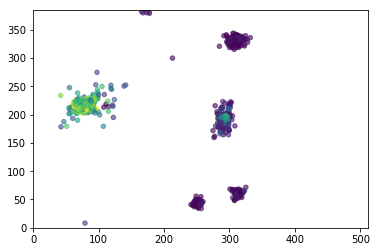/Users/amorriso/anaconda/lib/python3.6/site-packages/matplotlib/lines.py:1206: FutureWarning: comparison to None will result in an elementwise object comparison in the future.
if self._markerfacecolor != fc:

Check the DataFrame once, and then check it again after renaming the columns:
In :
df[:15]

Out:
x y filename fringe rx ry angle cluster
0 107.716469 213.009577 06240907_proc_00254.png 1.000000 85.034929 67.943204 -47.505782 0
1 114.698967 213.766703 06240907_proc_00258.png 1.333333 67.924027 67.389913 -51.659952 0
2 111.190662 218.375451 06240907_proc_00270.png 0.714286 67.455082 57.088226 -63.335567 0
3 113.800339 223.653310 06240907_proc_00276.png 8.333333 86.160744 73.501320 -73.822837 0
4 88.625250 218.599081 06240907_proc_00279.png 7.200000 119.292404 107.265178 -76.700412 0
5 81.290269 220.570363 06240907_proc_00281.png 7.333333 115.024131 109.400213 -91.981419 0
6 81.476925 215.762886 06240907_proc_00282.png 6.166667 115.916690 111.225947 -51.426068 0
7 72.502562 219.822452 06240907_proc_00292.png 7.200000 115.302500 108.964856 -54.631973 0
8 71.396729 213.876289 06240907_proc_00295.png 7.000000 132.873660 114.236231 -88.764995 0
9 73.012500 206.005209 06240907_proc_00299.png 10.000000 116.456652 113.427691 -82.312357 0
10 62.431250 206.850000 06240907_proc_00301.png 10.000000 104.117715 88.929126 -2.347311 0
11 141.296875 252.166667 06240907_proc_00301.png 3.666667 55.919208 29.365025 62.916449 -1
12 71.331521 212.055188 06240907_proc_00306.png 8.166667 122.378310 99.126123 -52.857932 0
13 71.714899 208.812385 06240907_proc_00307.png 8.666667 107.007787 98.573020 11.509674 0
14 286.998737 170.834790 06240907_proc_00307.png 1.200000 34.312887 32.881617 -0.016536 1
In :
labels

Out:
array([0, 0, 0, ..., 0, 1, 3])
These next two lines are the magic that connect the clusters identified by DBSCAN with the original classifications so that we can plot the fringe measurements for each cluster over time.
Finally figured this out by reading the question posted here: https://datascience.stackexchange.com/questions/29587/python-clustering-and-labels
In :
cluster=pd.Series(labels)
df["cluster"] = cluster

Rename the DataFrame columns:
In :
df = df.rename(index=str, columns={0: "x", 1: "y",2:"filename", 3:"fringe",4:"rx", 5:"ry",6:"angle"})

Assign each cluster its own variable:
In :
cluster0 = df[df['cluster']==0]
cluster1 = df[df['cluster']==1]
cluster2 = df[df['cluster']==2]
cluster3 = df[df['cluster']==3]
cluster4 = df[df['cluster']==4]
cluster5 = df[df['cluster']==5]
cluster6 = df[df['cluster']==6]
cluster7 = df[df['cluster']==7]

Make plots!!!
In :
plt.scatter(cluster0.index, cluster0.fringe)
plt.show()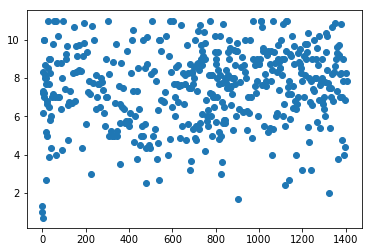In :
plt.scatter(cluster1.index, cluster1.fringe)
plt.show()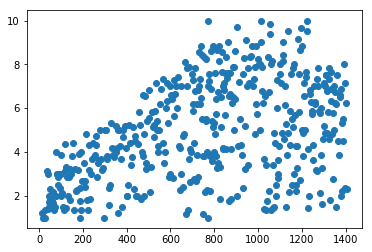In :
plt.scatter(cluster2.index, cluster2.fringe)
plt.show()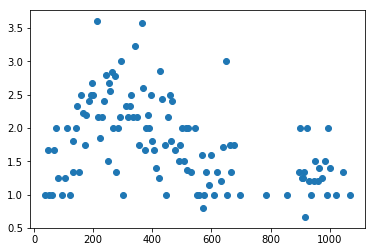In :
plt.scatter(cluster3.index, cluster3.fringe)
plt.show()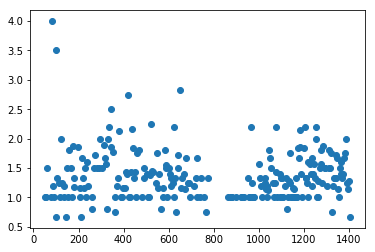In :
plt.scatter(cluster4.index, cluster4.fringe)
plt.show()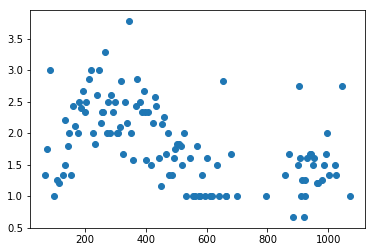In :
plt.scatter(cluster5.index, cluster5.fringe)
plt.show()